Dynamic Chiropractic – May 6, 2010, Vol. 28, Issue 10

## Biomechanically Correct Terms in Medical Writing

###### By Arthur Croft, DC, MS, MPH, FACO

The question of injury or legal causation is often central to the medicolegal question arising out of the motor vehicle collisions (MVC) most of us treat. This is sometimes referred to as the "special question" on jury instructions.

When the plaintiff fails to persuade jurors on this question, defense verdicts are often the fallout.

A physician's final or narrative report should address causation in some declarative form, such as, "It is my professional opinion that the MVC of [date] is the proximate and sole cause of the injuries and subsequent impairment(s) described above." In some cases, the physician may want to address opinions rendered by opposing experts or by an insurance claims adjustor. For example, the responsible insurer may have denied responsibility for medical bills, stating, that because the property damage to the plaintiff's car was not substantial, they don't feel the plaintiff would have been injured. This has become more or less pro forma for most insurers. However, the theory that a direct correlation exists between property damage and injury risk in the special context of collisions under 20 mph has been nullified in a large meta-analysis.1

In other cases, this "low crash injury probability" opinion may have been offered by an auto crash reconstructionist (ACR) or biomechanical expert to whose report you are privy. Following my recommendations, many doctors will often enter into a discussion of crash mechanics and/or biomechanics. ACR is really only a stepping stone to biomechanics, so in the context of MVC, the two overlap quite a bit, as do their respective phraseologies and lexicon. My observation is that doctors are sometimes unfamiliar with the precise definitions and use of this terminology, which can seriously detract from the credibility of the doctor's opinions. Let's review some of the terms that I see misused or misunderstood most often. They appear below in no particular logical order.

Speed, Velocity, and Acceleration

These terms are all related, but they have distinctly unique meaning and are definitively not interchangeable. Speed is a scalar quantity, meaning that it has only amplitude or magnitude. Your car's speedometer, for example, will give you your car's speed at any moment in time, but it has no information about your car's bearing or direction. Velocity, on the other hand, is a vector quantity: it has both magnitude and direction. In many physics applications, including ACR, we need to consider both features, so velocity is the better term. It allows us to deconstruct velocity into, for example, Cartesian components x and y in a 2-D setting, or x, y and z in 3-D.

Another way of looking at velocity is that it is the rate of change of position. In equation form, it is: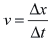where v is velocity, x is position in feet, and t is time in seconds. It can also be said to be the first derivative of position with respect to time. In the language of differential calculus this would be expressed as: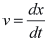The derivative becomes possible as a way of determining the instantaneous velocity once we have a function to use that relates position to time (e.g., x = x[t]). In a real sense, this is what your car's speedometer does while you are driving.

The term delta V is frequently used in ACR and biomechanics, usually with some erroneous connection to risk threshold, although that is a subject for another discussion. The delta V signifies the change of velocity and, although it is not the Holy Grail engineers make it out to be, it is an important crash metric. If one has stopped at a traffic signal and is struck suddenly from the rear by another vehicle, the car will lurch forward as a result of the impact. If the car had been initially fully stopped (v0=0 fps) and its subsequent highest post-impact velocity (v1) attained was 10 fps, its delta V would be 10 fps (v1-v0). Note that the delta V for the striking vehicle will be signed negatively because it will typically slow down as a result of the collision.

We often hear terms such as impact speed, collision speed or crash speed. These are imprecise terms because their real meaning may vary between users. For example, if one car is traveling in a southbound lane at 20 mph and runs into the rear of car in front of it, which is traveling in the same direction but at a slower speed of 10 mph, what is the impact speed (or collision speed or crash speed)? The answer depends on your definition of those terms; there are no standard definitions. The preferable terminology is closing velocity or differential velocity. In the above case, the closing velocity for these two vehicles would be 10 mph. If they were opposing each other in a head-on configuration, their closing velocity would be 30 mph.

While velocity is the rate of change of position with respect to time, acceleration is the rate of change of velocity with respect to time: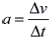where a is acceleration in fps2 (Vector quantities are usually written in bold). Acceleration can also be considered the second derivative of position with respect to time: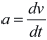Figure 1. The acceleration-time history for a crash test using a human volunteer in a 9 mph rear impact car-to-car test conducted at my institute. This plot demonstrates the difference between peak and mean acceleration, as well as the reproducibility of the various accelerometers used in the head array. Note that the delta V is plotted on the right abscissa. It is important to remember that equations 1 and 3 provide only average or mean values because we are essentially averaging the total change of position or velocity (numerator) with respect to the total duration of the position or velocity change (denominator). This can be very important when it come to forensic questions because the peak acceleration is typically two to three times higher in amplitude than mean acceleration. We are interested in the peak acceleration, not the mean, Figure 1.

ACRs and biomechanists frequently estimate mean values when working for the defense, because the values appear more benign. They also usually incorrectly assume that the occupant's acceleration and the vehicle's acceleration are the same. The occupant's peak head acceleration is typically two to four times that of the vehicle. Unfortunately, it is rare that the plaintiff's lawyer or treating doctors understand these important relationships.

Figure 2. This is the acceleration-time history of the same subject as in Figure 1, only in slightly lower velocity test. The rather unique risk envelope of the human occupant is illustrated here in that the vertical (z) acceleration pulse measured at the head is markedly higher than those of the vehicle or even the thorax. The net effect on the occupant is axial spinal compression. In a MVC, the occupants' heads will not only translate on a more or less horizontal path, they will also be accelerated in the z vector (vertically). In Figure 2, the effect of vertical acceleration is illustrated. In our SPECT study series, we speculated that end plate fractures were responsible for many patients' ongoing neck pain and these most likely occur during high peak compressive loads (about 210 msec in this plot).2

Forces and Moments

The concept of force derives from Newton's second law of motion in which he demonstrated that force, F, is equal to mass, m, times acceleration, a. Using metric units, it is expressed in Newtons, N. One Newton is the equivalent of about 0.225 lb (about the weight of an apple, as it turns out). Weight, however, is a unique kind of force that results from the effect of gravity. On the moon, your weight would be about 1/6 of what it is on Earth. Your mass, however, would be the same as it is on Earth. This is one reason why SI units are preferable to the U.S. Customary System (USCS). The Newton has, as its units, kilogram-meter/second2, whereas the British system uses slug-foot/second2. You sometimes see the term pound-force or lbf in the USCS, a force based on gravity at sea level. It is one pound.

When speaking of the forces that can be exerted on the human body, there are internal (such as muscular) and external forces (such as a blunt force or loads applied to the body). Deriving from Newton's second law of motion, sometimes referred to as the law of action and reaction, many forces are force systems comprising two or more forces. For example, when standing, we apply a vertical force to the ground through our feet as a result of gravity acting on the mass of our body. The ground exerts an equal but opposite force up through our feet. This ground reactive force is relevant also to all joints and opposes muscular forces applied to them. Normal forces are perpendicular to the orientation of the surface to which the load is applied. The ground reactive force just mentioned is normal to the ground's surface. Tangential forces occur when something is pushed horizontally along the ground, for example.

Forces can be coplanar, acting on a 2-D grid, or more complex. Collinear forces are those that share a common line of action, examples being a car rear-ending another car or two teams of people pulling on a rope in a tug of war. But these have other descriptive names as well. Axially compressing the spine is a compressive force. Stretching it produces tensile force. When a force moves perpendicular to a structure like the spine, it is a shear force. These shear forces are another major source of spinal injury and occur in collisions as a result of differential loads being applied to the head and torso in the case of the cervical spine. These can occur with or without head contact. Vertical loading, such as from a shallow dive injury, can also result in shear.

Bending produces forces called bending moments and twisting produces torque. A moment, M, is equal to the force, F, times the distance. Its units are Newton-meters (N-m) in SI units, or ft-lb in USCS units. Some of the important criteria for gauging injury risk are based on approximations of bending moments at the occipital condyles. Known as injury assessment reference values (IARVs), many of these have derivations that are dubious at best, and rest on assumptions about theoretical models of injury mechanisms that are out of date and incomplete, but that is also a subject for another editorial. Finally, there are mass moments of inertia, polar moments of inertia and area moments of inertia, which we'll leave for another editorial.

Degrees of Freedom

When I was in chiropractic school, I learned that bending the head to the side was called lateral flexion. Forward bending was simply flexion. Backward bending was extension. Turning to either side was rotation. Engineers usually think of extension as a linear elongation, such as when you extend a collapsible ladder or telescope. They often describe rotation as torque. In addition to bending, the spine also translates to some degree. Thus, it can bend around the x, y and z axes, and it can also (to a lesser degree) translate along those axes. We say it has, therefore, six degrees of freedom. Note that the six DOF is not the six (counting left and right) bending motions alone.

Inertia

Newton's first law of motion is sometimes called the law of inertia. This is the one that tells us that a body at rest will remain at rest unless it is acted upon by a net external force. The word net is important here because equal but opposite collinear forces would cancel and there would be no net force. The law also states that a body moving in uniform motion (i.e., straight line at constant velocity) will continue to do so unless, again, it is acted upon by a net or unbalanced force. When in uniform motion or stationary, the net force acting on a body must be zero, since there is no acceleration, and the object or body is said to be in equilibrium. Inertia is the resistance to the change in motion of matter, and mass is the quantitative measure of inertia.

Consider a person sitting in a stationary car that is struck from the rear. Initially, the car will accelerate forward while the person's inertia resists that forward motion. As the car continues forward, the torso is secondarily accelerated. Even as the torso begins to accelerate and translate forward, the head's inertia holds it in space, resisting the forward motion until the elastic forces generated in the neck, and perhaps also the forward-moving head restraint, supply the unbalanced net force needed to accelerate the head. As a result, we see the car's acceleration first, followed by the torso's, and then the head's (Figure 2).

Impulse

The impulse is another important quantitative measure of injury risk that indirectly considers the anisotropic, non-linearly elastic, viscous and time-dependent material behavior called visco-elasticity. It is a function of force and time. This is critically important for visco-elastic tissues such as muscle, ligament, joint capsule, nerve, disc or even bone, which are usually more vulnerable to the high rate of loading as seen in Figure 2, where the major element of compression in the spine occurs in the 100 msec (0.1 second) between 150 and 250 msec. Newton's second law of motion can be reformulated to introduce the impulse-momentum theorem, which is a relationship I use commonly in biomechanical analyses. It can be applied to just about any temporal loading condition. It works this way: Newton's second law of motion is the relationship between force, acceleration, and mass: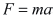Linear momentum, p, is the vector mv, or the product of mass and velocity: p=mv.

The idea here is to consider a loading event between two points in time. Thus, there are two components to the relationship: a linear impulse of force, F, and the linear momentum change, or delta p, which is p2-p1. Integrating between these two times, we get the impulse-momentum theorem: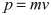In less formal terms, the definite integral on the left side represents the linear impulse of force, F, in the time domain (i.e., delta t = t2-t1) and this simplifies to: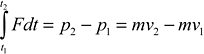In the same way that we can integrate the area under the acceleration curve to obtain delta V, the impulse can be determined by integrating the area under the curve representing the magnitude and direction of the force between t2 and t1. For practical purposes, in these high-velocity crashes, the impulse loading interval is so brief that it can be assumed to have a constant force, F, and can thus be reduced further to: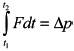The net force over this interval can then be determined by rearranging Equation 8: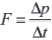As a demonstration of what one can do with the impulse-momentum theorem, let's use an example of a case I had some time back in which a man injured his right elbow in a frontal crash in which he was the right front-seat passenger. I won't go into the details of how it was determined, but the loading phase in this case was assumed to be 30 msec (0.03 second) and the delta V was assumed to be 31.8 mph (14.2 m/s). He weighed 198 lb (90 kg). The momentum then would be: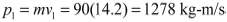Plugging the results into Equation 9, we get: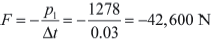Notice that because the velocity at t2 would have been zero (he came to an abrupt stop in the crash), p2 (mv) would have been zero, so it drops out of the equation. Since the relationship called for p2-p1, p1 will be signed negatively. This force is equal to -9,576 lb (or lbf). Considering the dissipative force potentially produced by bracing against the floor pan, which would anchor the pelvis, the effective mass value in equation 10 was halved so that I was considering only the momentum of the torso, upper extremities, and head, but it still implies a brief impulse of more than two tons of force going into the elbow.

References

1. Croft AC, Freeman MD. Correlating crash severity with injury risk, injury severity, and long-term symptoms in low velocity motor vehicle collisions. Med Sci Monit, 2005;11:RA316-21.
2. Freeman MD, Sapir D, Boutselis A, et al. Whiplash injury and occult vertebral fracture: a case series of bone SPECT imaging of patients with persisting spine pain following a motor vehicle crash. Cervical Spine Research Society 29th Annual Meeting. Monterey, Calif., 2001.

Click here for previous articles by Arthur Croft, DC, MS, MPH, FACO.